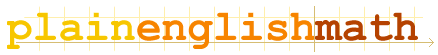About this site Fundamentals Vectors Matrices Circles and Triangles Complex Numbers Sequences and Series Calculus Appendices Glossary . . .You are here: MathematicsFundamentals → Fundamentals

Fundamentals

Numbers

Algebra and Equations
and how to solve them.

Relations
Equations are only one type of mathematical relation. You've met the = sign. Now meet its relatives...

Functions
Variables that depend on other variables...

Sets
Sets of numbers or mathematical values

The greek alphabet
Every mathematician uses it!